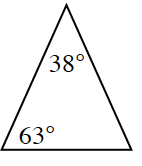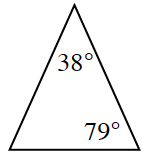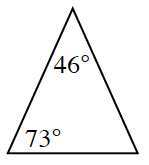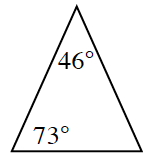### Home > GC > Chapter 3 > Lesson 3.1.4 > Problem3-39

3-39.

In recent lessons, you have learned that similar triangles have equal corresponding angles. Is it possible to have equal corresponding angles when the triangles do not appear to match? What if you are not given all three angle measures? Consider the two cases below.

1. Find the measure of the third angle in the first pair of triangles below. Compare the two triangles. What do you notice?

All angles in a triangle add to $180º$.2. Examine the second pair of triangles below. Without calculating, do you know that the unmarked angles must be equal? Why or why not?

The unmarked angles are equal. Why?